# I.E. Irodov Solutions on Optics Of Moving Sources

The solutions of the Problems In General Physics I.E. Irodov - Optics of Moving Sources are given on this page. As far as any entrance exam is concerned, optics is an important topic. Doppler effect and Vavilov-Cherenkov effect are the two main topics in optics of moving sources. Students can expect one question from optics for any entrance test. Students are recommended to go through these solutions so that they can understand the pattern and type of questions asked from this topic.

Learning these solutions will help students to achieve higher ranks in the JEE exam and other competitive exams.

### I.E. Irodov Solutions on Optics Of Moving Sources

1. In the Fizeau experiment on measurement of the velocity of light the distance between the gear wheel and the mirror is l = 7.0 km, the number of teeth is z = 720. Two successive disappearances of light are observed at the following rotation velocities of the wheel: n1 = 283 rps and n2 = 313 rps. Find the velocity of light.

Solution:

1. In the Fizean experiment, light disappears when the wheel rotates to bring a tooth in the position formerly occupied by a gap in the time taken by light to go from the wheel to the mirror and back. Thus distance travelled = 2l. Suppose the mth tooth after the gap has come in place of the latter. Then time taken = 2(m - 1) + 1)/(2zn1) sec in the first case

= (2m + 1)/2zn2 sec in the second case = 1/z(n2 - n1)

Then c = 2lz(n2 - n1)

= 3.024×108 m/sec

2. A source of light moves with velocity v relative to a receiver. Demonstrate that for v << c the fractional variation of frequency of light is defined by Eq. ∆ω/ω = (v/c)cos θ

Solution:

1. When v << c time dilation effect of relativity can be neglected (i.e. t’ ≈ t ) and we can use time in the reference frame fixed to the observer. Suppose the source emits short pulses with intervals T0. Then in the reference frame fixed to the receiver the distance between two seccessive pulses is λ = cT0 - vrT0 when measured along the observation line. Here vr = v cos θ is the projection of the source velocity on the observation line. The frequency of the pulses received by the observer is

v = c/λ

= v0/1 - vz/c

≈ v0(1 + vr/c)

(The formula is accurate to first order only)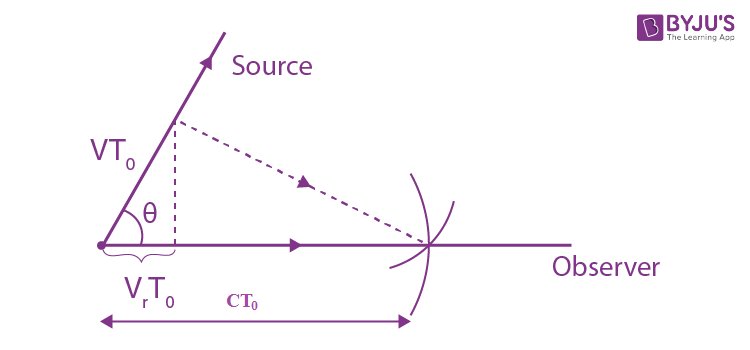Thus (v - v0)/v0 = vr/c = v cos θ/c

The frequency increases when the source is moving towards the observer.

3. One of the spectral lines emitted by excited He+ ions has a wavelength λ = 410 nm. Find the Doppler shift ∆λ of that line when observed at an angle 0 = 300 to the beam of moving ions possessing kinetic energy T = 10 MeV.

Solution:

1.∆v/v = (v/c)cos θ from the previous problem.

But vλ = c gives a differentiation

∆v/v = -∆λ/λ

So ∆λ = -λ√(v2/c2) cos θ

= -λ√(2T/mc2) cos θ

On using T = ½ mv2, m = mass of He+ ion

We use mc2 = 4×938 MeV. Putting other values

∆λ = -26 nm

4. When a spectral line of wavelength X = 0.59 [tm is observed in the directions to the opposite edges of the solar disc along its equator, there is a difference in wavelengths equal to off, = 8.0 pm. Find the period of the Sun's revolution about its own axis.

Solution:

1. One end of the solar disc is moving towards us while the other end is moving away from us. The angle θ between the direction in which the edges of the disc are moving and the line of observation is small. (cos θ ≈ 1). Thus

∆λ/λ = 2ωR/c

where ω = 2π/T is the angular velocity of the Sun. Thus

ω = c∆λ/2Rλ

So T = 4πRλ/c∆λ

Putting the values (R = 6.95×108m)

we get T = 24.85 days.

5. The Doppler effect has made it possible to discover the double stars which are so distant that their resolution by means of a telescope is impossible. The spectral lines of such stars periodically become doublets indicating that the radiation does come from two stars revolving about their centre of mass. Assuming the masses of the two stars to be equal, find the distance between them and their masses if the maximum splitting of the spectral lines is equal to (∆λ/λ)m = 1.2.10-4 and occurs every T = 30 days.

Solution:

1. Maximum splitting of the spectral lines will occur when both of the stars are moving in the direction of line of observation as shown.We then have the equations

(∆λ/λ)m = 2v/c

mv2/R = γm2/4R2

T = πR/v

From these we get

d = 2R = (∆λ/λ)m

= cT/π

= 2.97×107 km

m = (∆λ/λ)mc3T/2πγ

= 2.9×1029 kg

6. A plane electromagnetic wave of frequency ω0 falls normally on the surface of a mirror approaching with a relativistic velocity V. Making use of the Doppler formula, find the frequency of the reflected wave. Simplify the obtained expression for the case V << c.

Solution:

1. We define the frame S (the lab frame) by the condition of the problem. In this frame the mirror is moving with velocity v (along say x axis) towards left and light of frequency ω0 is approaching it from the left. We introduce the frame S’ whose axes are parallel to those of S but which is moving with velocity v along x axis towards left (so that the mirror is at rest in S'). In S' the frequency of the incident light is ω1 = ω0[(1 + v/c)/(1 - v/c)]1/2

In S.' the reflected light still has frequency ω1 but it is now moving towards left. When we transform back to S this reflected light has the frequency

ω = ω1[(1 + v/c)/(1 - v/c)]1/2

= ω0[(1 + v/c)/(1 - v/c)]

In the nonrelativistic limit

ω ≈ ω0(1 + 2v/c)

7. A radar operates at a wavelength λ = 50.0 cm. Find the velocity of an approaching aircraft if the beat frequency between the transmitted signal and the signal reflected from the aircraft is equal to ∆v = 1.00 kHz at the radar location.

Solution:

1. From the previous problem, the beat frequency is clearly

∆v = v0(2v/c)

= 2v/(c/v0)

= 2v/λ0

Hence v = ½ λ∆v

= (103/2)×50 cm/sec

= 900 km/hour

8. Taking into account that the wave phase ωt - kx is an invariant, i.e. it retains its value on transition from one inertial frame to another, determine how the frequency ω and the wave number k entering the expression for the wave phase are transformed. Examine the unidimensional case.

Solution:

1. From the invariance of phase under Lorentz transformations we get

ωt - kx = ω’t’ - k’x’

Here ω = ck.

The primed coordinates refer to the frame S’ which is moving to the right with velocity v:

Then x’ = γ(x - vt)

t’ = γ(t - vx/c2)

Where γ = √(1 - v2/c2)-1

Substituting and equating the coefficients of t and x.

ω = γω’ + γk’v

= ω’ (1 + v/c)/√(1 - v2/c2)

k = γω’v/c2 + γk’

= k’(1 + v/c)/√(1 - v2/c2)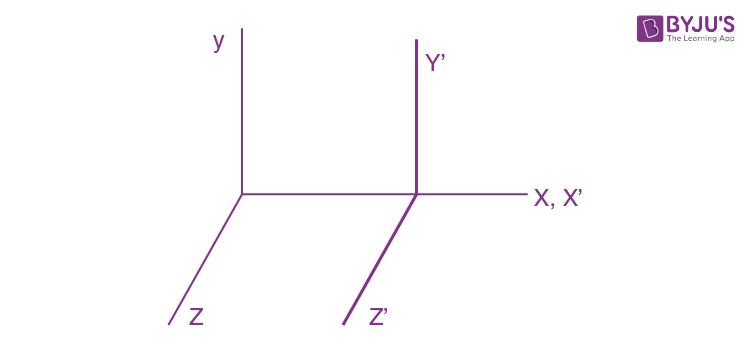9. How fast does a certain nebula recede if the hydrogen line λ = 434 nm in its spectrum is displaced by 130 nm toward longer wavelengths?

Solution:

1. From the previous problem using k = 2π/λ

We get λ’ = λ√[(1+ v/c)/(1 - v/c)]

Thus (1 + v/c)/(1 - v/c) = λ’22

Or v/c = (λ’2- λ2)/(λ’22)

= (5642 - 4342)/( 5642 + 4342)

= 0.256

10. How fast should a car move for the driver to perceive a red traffic light (λ≈ 0.70 μm) as a green one (λ’≈ 0.55 μm)?

Solution:

1. As in the previous problem v/c = (λ2 - λ’2)/(λ2 +λ’2)

So v = c[(λ/λ’)2 - 1]/[(λ/λ’)2 + 1]

= 7.1×104 km/s

11. An observer moves with velocity v1= ½ c along a straight line. In front of him, a source of monochromatic light moves with velocity v2= ½ c in the same direction and along the same straight line. The proper frequency of light is equal to ω0. Find the frequency of light registered by the observer.

Solution:

1. We go to the frame in which the observer is at rest. In this frame the velocity of the source of light is, by relativistic velocity addition formula

V=\frac{\frac{3}{4}c-\frac{1}{2}c}{1-(\frac{\frac{3}{4}c\times\frac{1}{2}c}{c^{2}})}=\frac{2c}{5}

When this source emits light of proper frequency ω0 , the frequency recorded by observer will be

\omega =\omega _{0}\sqrt{\frac{1-\frac{v}{c}}{1+\frac{v}{c}}+\frac{v}{c}}=\sqrt{\frac{3}{7}}\omega _{0}

Note that ω < ωo as the source is moving away from the observer (red shift).

12. One of the spectral lines of atomic hydrogen has the wavelength λ =656.3 nm. Find the Doppler shift Δλ of that line when observed at right angles to the beam of hydrogen atoms with kinetic energy T = 1.0 MeV (the transverse Doppler effect).

Solution:

1. In transverse Dopler effect

\omega =\omega _{0}\sqrt{1-\beta ^{2}}=\omega _{0}(1-\frac{1}{2}\beta^{2})

\lambda =\frac{c}{\omega }=\frac{c}{\omega _{0}}(1+\frac{1}{2}\beta^{2})=\lambda _{0}(1+\frac{1}{2}\beta^{2})

Hence, \Delta \lambda=\frac{1}{2}\beta^{2}\lambda

\beta^{2}=\frac{v^{2}}{c^{2}}=\frac{2T}{mC^{2}}

Where T= KE of H atoms

\Delta \lambda=\frac{T}{mC^{2}}\lambda =\frac{1}{938}\times 656.3nm=0.70 nm

13. A source emitting electromagnetic signals with proper frequency ω0= 3.0 x10 10 s-1 moves at a constant velocity v = 0.80 c along a straight line separated from a stationary observer P by a distance l (Fig. 5.37). Find the frequency of the signals perceived by the observer at the moment when

(a) the source is at the point O;

(b) the observer sees it at the point O.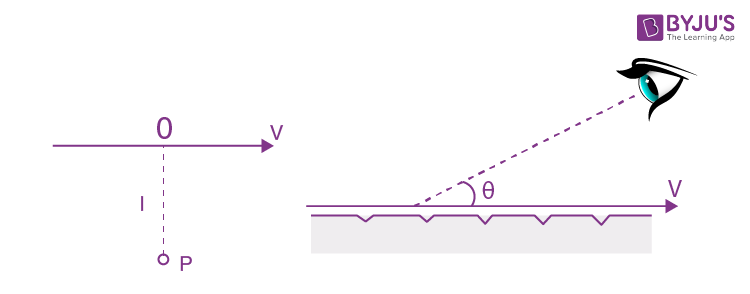Solution:

1. (a) If light is received by the observer at P at the moment when the source is at O, it must have been emitted by the source when it was at O' and travelled along O’P.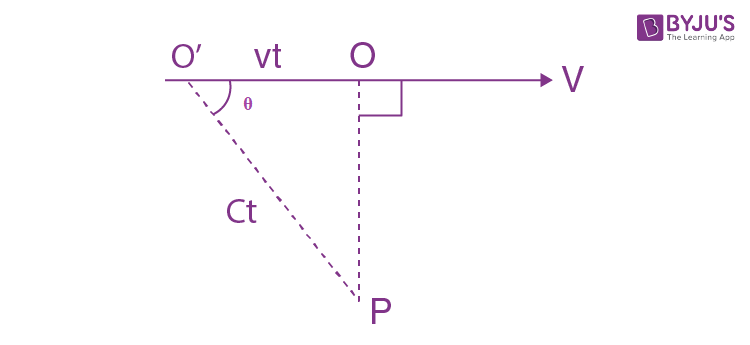Then if O’P = c t, then O’O = vt

And cos θ= v/c = β

In the frame of the observer, the frequency of the light is ω while its wave vector is

w/c (cos θ, sin θ, 0)

we can calculate the value of ω by relating it to proper frequency ω. The relation is

\omega _{0}= \frac{\omega}{\sqrt{1-\beta ^{2}}}=(1-\beta \cos \Theta )

To derive the formula is this form it is easiest to note that

\frac{\omega}{\sqrt{1-\frac{v}{c^{2}}}}-\frac{\vec{k}\vec{v}}{\sqrt{1-\frac{v}{c^{2}}}}

Is an n variant which takes the value ω0 in the rest frame of the source.

\omega =\frac{\omega _{0}\sqrt{1-\beta ^{2}}}{\sqrt{1-\beta ^{2}}}=\frac{\omega _{o}}{\sqrt{1-\beta ^{2}}}=5.9\times10^{-10}sec^{-1}

(b) For the light to be is an received at the instant observer sees the source at O, light must be emitted when the observer is at O at 900 = θ

cos θ = 0

\omega _{0}= \frac{\omega }{\sqrt{1-\beta ^{2}}}

\omega= {\omega_{o} }{\sqrt{1-\beta ^{2}}}= 1.8 \times 10^{10} sec^{-1}

In this case the observer will receive light along OP and he will “see” that the source is at O even though the source will have moved ahead at the instant the light is received.

14. A narrow beam of electrons passes immediately over the surface of a metallic mirror with a diffraction grating with period d = 2.0 μm inscribed on it. The electrons move with velocity v, comparable to c, at right angles to the lines of the grating. The trajectory of the electrons can be seen in the form of a strip, whose colouring depends on the observation angle θ. Interpret this phenomenon. Find the wavelength of the Ans. radiation observed at an angle θ = 450

Solution:

1. An electron moving in front of a metal mirror sees an image change of equal and opposite type. The two together constitute a dipole. Let us look at the problem in the rest frame of the electron. In this frame the grating period is Lorenz contracted to

d= d\sqrt{1-\frac{v^{2}}{c^{2}}}

Because the metal has etchings the dipole moment of electron distuibed with a period d’/v

The corresponding frequency is image pair is periodically v/d’ which is also the proper frequency of radiation emitted. Due to Doppler effect the frequency observed at an angle 0 is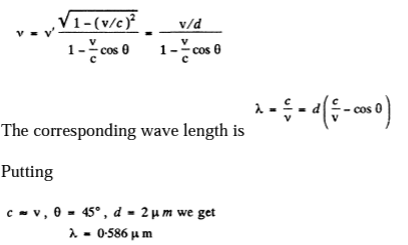15. A gas consists of atoms of mass m being in thermodynamic equilibrium at temperature T. Suppose ωo is the natural frequency of light emitted by the atoms.

(a) Demonstrate that the spectral distribution of the emitted light is defined by the formula Iw= Ioe-a(1-w/w0)2

(Io is the spectral intensity corresponding to the frequency ωo , a = mc2/ 2KT)

(b)Find the relative width Δω/ω0 of a given spectral line, i.e. the width of the line between the frequencies at which Iw = Io/2

Solution:

1. (a) Let vx be the projection of the velocity vector of the radiating atom on the observation direction. The number of atoms with projections falling within the interval vx and

vx + dvx

n(vx )dvx ∼ exp (-mVx2/ 2KT)dVx

The frequency of light emitted by the atoms moving with velocity vx is

ω = ωo( 1+vx/ c)

From the expressions the frequency distribution of atoms can be found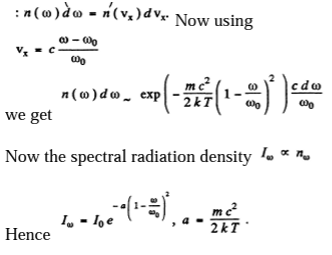The constant of proportionality is fixed by Io.

(b)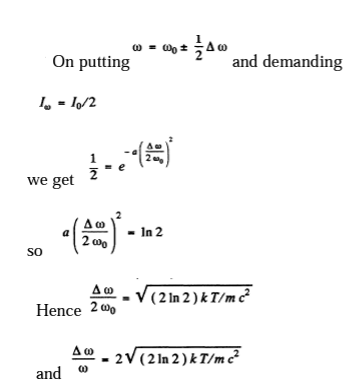16. A plane electromagnetic wave propagates in a medium moving with constant velocity V ≪ c relative to an inertial frame K. Find the velocity of that wave in the frame K if the refractive index of the medium is equal to n and the propagation direction of the wave coincides with that of the medium.

Solution:

1. In vacuum inertial frames are all equivalent; the velocity of light is c in any frame. This equivalence of inertial frames does not hold in material media and here the frame in which the medium is at rest is singled out. It is in this frame that the velocity of light is c/n where n is the refractive index of light for that medium. The velocity of light in the frame in which the medium is moving is then by the law of addition of velocities.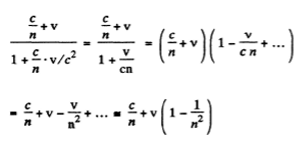This is the velocity of light in the medium in a frame in which the medium is moving with velocity v « c .

17. Aberration of light is the apparent displacement of stars attributable to the effect of the orbital motion of the Earth. The direction to a star in the ecliptic plane varies periodically, and the star performs apparent oscillations within an angle δθ = 41". Find the orbital velocity of the Earth.

Solution:

1. Although speed of light is the same in all inertial frames of reference according to the principles of relativity, the direction of a light ray can appear different in different frames. This phenomenon is called aberration and to first order in v/c, can be calculated by the elementary law of addition of velocities applied to light waves. The angle of aberration is tan-1 v/c.

and in the present case ½ δθ on either side. On equating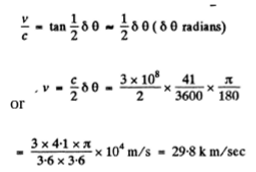18. Demonstrate that the angle θ between the propagation direction of light and the x axis transforms on transition from the reference frame K to K' according to the formula

\cos \Theta '=\frac{\cos \Theta -\beta }{1-\beta \cos \Theta }

where β = V/c and V is the velocity of the frame K' with respect to the frame K. The x and x' axes of the reference frames coincide.

Solution:

1. We consider the invariance of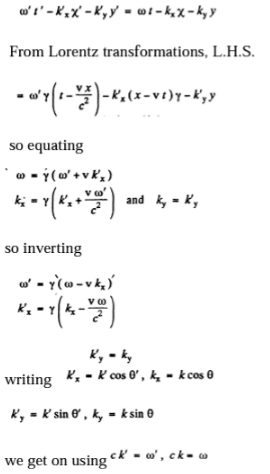\cos \Theta '=\frac{\cos \Theta -\beta }{1-\beta \cos \Theta }

Where β= v/c and the printed frame is moving with velocity v in the x direction with respect to the unprimed frame. For small β << 1 the situation is as shown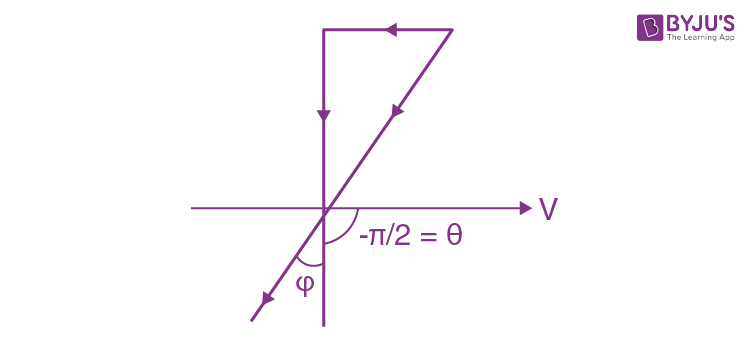We see that cosθ’= -β

If θ= -π/2

Then

\Theta '=-(\frac{\pi }{2}+\arcsin \beta )

This is exactly what we get from elementary velocities, non relativistic law of addition of velocities.

19. Find the aperture angle of a cone in which all the stars located in the semi sphere for an observer on the Earth will be visible if one moves relative to the Earth with relativistic velocity V differing by 1.0% from the velocity of light. Make use of the formula of the foregoing problem.

Solution:

1. The statement of the problem is not quite properly worked and is in fact misleading. The correct situation is described below. We consider, for simplicity, stars in the x z plane. Then the previous formula is applicable, and we have

\cos \theta '=\frac{\cos \theta-\beta }{1-\beta \cos \theta}=\frac{\cos \theta -0.99 }{1- 0.99 \cos \theta}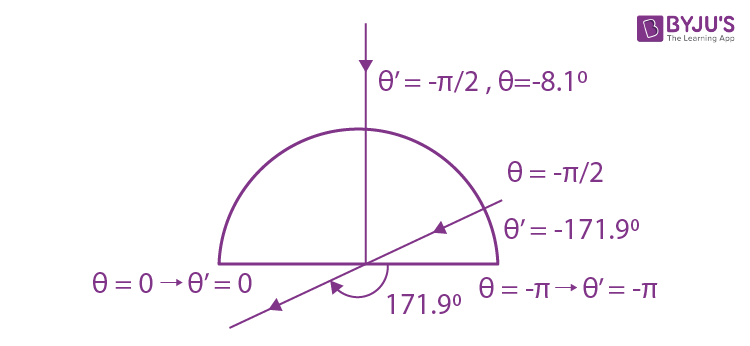The distribution of θ' is given in the diagram below The light that appears to come from the forward quadrant in the frame K (θ = -π to (θ = -π/2) , is compressed into an angle of magnitude + 8.1° in the forward direction while the remaining stars are spread out. The three dimensional distribution can also be found out from the three dimensional generalization of the formula in the previous problems.

20. Find the conditions under which a charged particle moving uniformly through a medium with refractive index n emits light (the VavilovCherenkov effect). Find also the direction of that radiation. Instruction. Consider the interference of oscillations induced by the particle at various moments of time.

Solution:

1. The field induced by a charged particle moving with velocity V excites the atoms of the medium turning them into sources of light waves. Let us consider two arbitrary points A and B along the path of the particle. The light waves emitted from these points when the particle passes them reach the point P simultaneously and reinforce each other provided they are in phase which is the case is general if the time taken by the light wave to propagate from the point A to the point C is equal to that taken by the particle to fly over the distance AB. Hence we obtain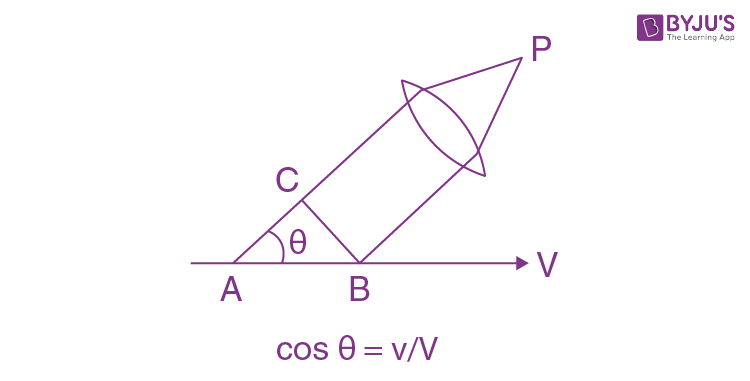Where v = c/n is the phase velocity of light. It is evident that the radiation is possible only if V >v i.e. when the velocity of the particle exceeds the phase velocity of light in the medium.